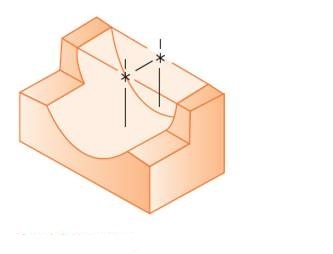Chapter 16, Problem 8P

Chapter
Section
Textbook ProblemTo determine

Draw the top, the front and the right side orthogonal views of the given object and indicate when an object needs only one or two views.

Explanation

Calculation:

Sketch the given Figure as follows,

When the observer observes the object from the front side, the view captured on the front plane is represented below Figure 2.

When the observer observes the object from the top side, the view captured on the top plane is represented below in Figure 3.

Refer to Figure 3, the center of lines which shows where the center of holes or centers of cylinders are present in the object...

Still sussing out bartleby?

Check out a sample textbook solution.

See a sample solution

The Solution to Your Study Problems

Bartleby provides explanations to thousands of textbook problems written by our experts, many with advanced degrees!

Get Started

Find more solutions based on key concepts